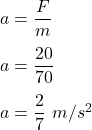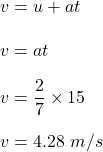Question

19. After a snowstorm, you put on your frictionless skis and tie a rope to the back of your friend’s truck. Your total mass is 70 kg and the truck exerts a constant force of 20 N. How fast will you be going after 15 seconds, in m/s and MPH?

1.Explanation:

It is given that,

Total mass is 70 kg

The truck exerts a constant force of 20 N.

Then the net force is given by :

F = ma

a is acceleration of riderInitial velocity of rider is 0. So, using equation of kinematics to find the final velocity as :Since, 1 m/s = 2.23 mph

4.28 m/s = 9.57 mph

So, the speed of the rider is 4.28 m/s or 9.57 mph.